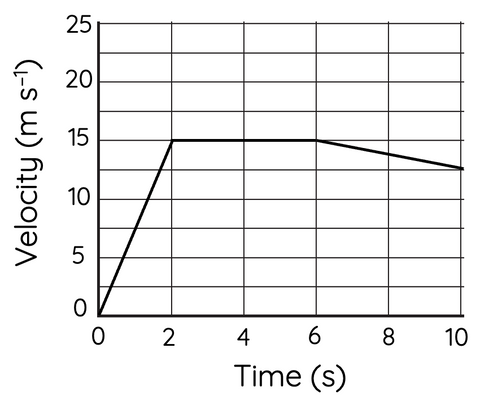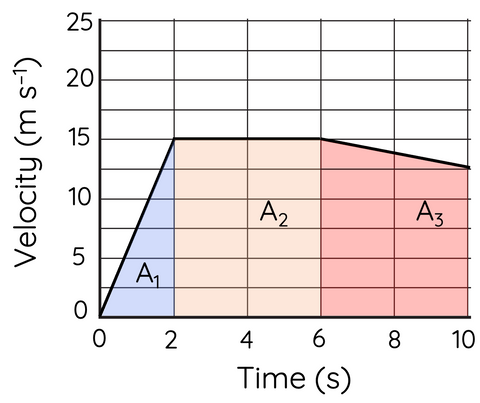# Instantaneous and Average Velocity

This topic is part of the HSC Physics course under the section Motion in a Straight Line.

### HSC Physics Syllabus

• conduct a practical investigation to gather data to facilitate the analysis of instantaneous and average velocity through:
– quantitative, first-hand measurements
– the graphical representation and interpretation of data (ACSPH061)

### How to Calculate Instantaneous and Average Velocity

Velocity is a fundamental concept in physics that helps us understand the motion of objects. It is defined as the rate at which an object changes its position with respect to time. In this article, we will explore two important types of velocity: instantaneous velocity and average velocity.

### What is Instantaneous Velocity?

Instantaneous velocity is the velocity of an object at a specific moment in time. For objects in motion with acceleration, the instantaneous velocity changes with time. When there is no acceleration, instantaneous velocity remains constant with time.

In simpler terms, instantaneous velocity is the velocity of an object at a particular instant in time. It tells us how fast an object is moving and in what direction at a specific point in time. For example, if a car is traveling at 60 km/h at 3:00 pm, then its instantaneous velocity at 3:00 pm is 60 km/h.

Finding instantaneous velocity on velocity vs time graph

To find the instantaneous velocity at a specific point on the velocity vs time graph, you need to read the value of the velocity at that point on the graph. The value of the velocity at a specific point on the graph represents the instantaneous velocity at that point.For example, in the velocity vs time graph above, the instantaneous velocity at = 4 s is 15 m/s, at = 10 s is 12.5 m/s.

For times when the instantaneous velocity cannot be precisely read off the graph, it can be calculated using the equation:

$$v=u+at$$

The acceleration in the first 2 seconds is given by

$$15=0+a\times 2$$

$$a=7.5 \hspace{1mm} m \hspace{1mm} s^{-2}$$

The instantaneous velocity at t = 1.2 s is given by

$$v=0+7.5 \times 1.2$$

$$v= 9 \hspace{1mm} m \hspace{1mm} s^{-1}$$

Finding instantaneous velocity using displacement time graph

To find the instantaneous velocity at a specific point on the displacement vs time graph, you need to calculate the slope of the tangent line at that point. The slope of the tangent line represents the instantaneous velocity at that point.For example, on the displacement vs time graph above, the instantaneous velocity at = 3 s can be determined by calculating the slope at = 3. The gradient is given by:

$$gradient = \frac{rise}{run}$$

$$gradient = \frac{80-0}{5-1.5}$$

$$gradient = 23 \hspace{1mm} m \hspace{1mm} s^{-1}$$

Therefore, the instantaneous velocity at ﻿t = ﻿3 s is 23 m/s.

### What is Average Velocity?

Average velocity is the average rate at which an object changes its position over a given time interval. It is calculated by dividing the displacement of the object by the time taken to travel that distance. Mathematically, we can represent average velocity as:

$$v_{average}=\frac{s}{t}$$

where v is the average velocity, is the displacement of the object, and t is the time taken to travel that distance.

In simpler terms, average velocity tells us the average speed and direction of an object over a given time interval. For example, if a car travels a distance of 120 km from 3:00 pm to 4:00 pm, then its average velocity over that time interval is 120 km/h.

To find the average velocity of an object over a given time interval, you need to calculate the displacement travelled during that interval, then divide it by the time taken.For example, the average velocity in the displacement vs time graph above is given by:

$$v_{average} = \frac{80}{5}$$

$$v_{average}= 16 \hspace{1mm} m \hspace{1mm} s^{-1}$$

To calculate the average velocity on a velocity vs time graph, the displacement must first be calculated from the area under the graph.For example, the area under the velocity vs time graph above is given by:

$$A_1 = \frac{1}{2}(2)(15)=15$$

$$A_2 = 15 \times (6-2) = 60$$

$$A_3 = \frac{1}{2}(10-6)(15+12.5) = 55$$

$$A_{total}=15+60+55=130 \hspace{1mm} m$$

Therefore, the displacement is 130 m.

The average velocity is given by:

$$v_{average} = \frac{s}{t}$$

$$v_{average} = \frac{130}{10}$$

$$v_{average} = 13 \hspace{1mm} m \hspace{1mm} s^{-1}$$